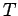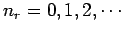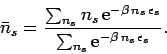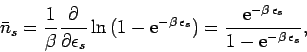Next: Bose-Einstein statistics Up: Quantum statistics Previous: Fermi-Dirac statistics

## Photon statistics

Up to now, we have assumed that the number of particlescontained in a given system is a fixed number. This is a reasonable assumption if the particles possess non-zero mass, since we are not generally considering relativistic systems in this course. However, this assumption breaks down for the case of photons, which are zero-mass bosons. In fact, photons enclosed in a container of volume, maintained at temperature, can readily be absorbed or emitted by the walls. Thus, for the special case of a gas of photons there is no requirement which limits the total number of particles.

It follows, from the above discussion, that photons obey a simplified form of Bose-Einstein statistics in which there is an unspecified total number of particles. This type of statistics is called photon statistics.

Consider the expression (584). For the case of photons, the numbersassume all valuesfor each, without any further restriction. It follows that the sumsin the numerator and denominator are identical and, therefore, cancel. Hence, Eq. (584) reduces to(598)

However, the above expression can be rewritten(599)

Now, the sum on the right-hand side of the above equation is an infinite geometric series, which can easily be evaluated. In fact,(600)

Thus, Eq. (599) gives(601)

or(602)

This is known as the Planck distribution, after the German physicist Max Planck who first proposed it in 1900 on purely empirical grounds.

Equation (583) can be integrated to give(603)

where use has been made of Eq. (602).Next: Bose-Einstein statistics Up: Quantum statistics Previous: Fermi-Dirac statistics
Richard Fitzpatrick 2006-02-02